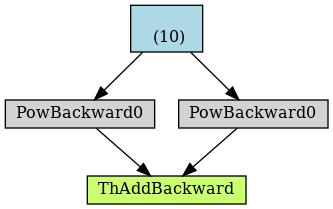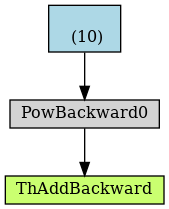In order to enable automatic differentiation, PyTorch keeps track of all operations involving tensors for which the gradient may need to be computed (i.e., require_grad is True). The operations are recorded as a directed graph. The detach() method constructs a new view on a tensor which is declared not to need gradients, i.e., it is to be excluded from further tracking of operations, and therefore the subgraph involving this view is not recorded.

This can be easily visualised using the torchviz package. Here is a simple fragment showing a set operations for which the gradient can be computed with respect to the input tensor x.

``````x=T.ones(10, requires_grad=True)

y=x**2
z=x**3
r=(y+z).sum()
make_dot(r).render("attached", format="png")
``````

The graph inferred by PyTorch is this:This program can be correctly differentiated to obtain the gradient:

``````>>> r.backward()
tensor([5., 5., 5., 5., 5., 5., 5., 5., 5., 5.])
``````

However if a detach is called then subsequent operations on that view will not be tracked. Here is a modification to the above fragment:

``````y=x**2
z=x.detach()**3
r=(y+z).sum()
make_dot(r).render("detached", format="png")
``````

Note that x is detached before being used in computation of z. And this is the graph of this modified fragment:As can be seen the branch of computation with x**3 is no longer tracked. This is reflected in the gradient of the result which no longer records the contribution of this branch:

``````>>> r.backward()